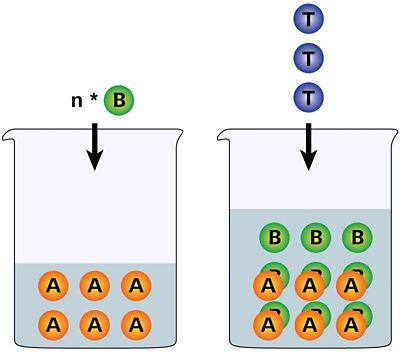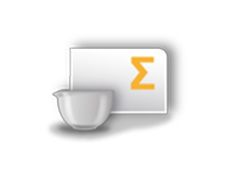You have been redirected to your local version of the requested page

#### Titrations can be classified in various ways: by their chemical reaction (e.g., acid-base titration or redox titration), the indication method (e.g., potentiometric titration or photometric titration), and last but not least by their titration principle (direct titration or indirect titration). In this article, I want to elaborate on a specific titration principle – the back-titration – which is also called «residual titration». Learn more about when it is used and how you should calculate results when using the back-titration principle.Figure 1. Reaction principle of a back-titration: Reagent B is added in excess to analyte A. After a defined waiting period which allows for the reaction between A and B, the excess of reagent B is titrated with titrant T.

### What is a back-titration?

In contrast to direct titrations, where analyte A directly reacts with titrant T, back-titrations are a subcategory of indirect titrations. Indirect titrations are used when, for example, no suitable sensor is available or the reaction is too slow for a practical direct titration.

During a back-titration, an exact volume of reagent B is added to the analyte A. Reagent B is usually a common titrant itself. The amount of reagent B is chosen in such a way that an excess remains after its interaction with analyte A. This excess is then titrated with titrant T. The amount of analyte A can then be determined from the difference between the added amount of reagent B and the remaining excess of reagent B.

As with any titration, both involved reactions must be quantitative, and stoichiometric factors involved for both reactions must be known.

### When are back-titrations used?

Back titrations are mainly used in the following cases:

• if the analyte is volatile (e.g., NH3) or an insoluble salt (e.g., Li2CO3)
• if the reaction between analyte A and titrant T is too slow for a practical direct titration
• if weak acid – weak base reactions are involved
• when no suitable indication method is available for a direct titration

Typical examples are complexometric titrations, for example aluminum with EDTA. This direct titration is only feasible at elevated temperatures. However, adding EDTA in excess to aluminum and back-titrating the residual EDTA with copper sulfate allows a titration at room temperature. This is not only true for aluminum, but for other metals as well.

Learn which metals can be titrated directly, and for which a back-titration is more feasible in our free monograph on complexometric titration.

Monograph: Complexometric (chelatometric) Titrations

Other examples include the saponification value and iodine value for edible fats and oils. For the saponification value, ethanolic KOH is added in excess to the fat or oil. After a determined refluxing time to saponify the oil or fat, the remaining excess is back-titrated with hydrochloric acid. The process is similar for the iodine value, where the remaining excess of iodine chloride (Wijs-solution) is back-titrated with sodium thiosulfate.

For more information on the analysis of edible fats and oils, take a look at our corresponding free Application Bulletin AB-141.Figure 2. Example of a Titrator equipped with an additional buret for the addition of reagent B.

### How is a back-titration performed?

A back-titration is performed according to the following general principle:

1. Add reagent B in excess to analyte A.
2. Allow reagent B to react with analyte A. This might require a certain waiting time or even refluxing (e.g., saponification value).
3. Titration of remaining excess of reagent B with titrant T.

For the first step, it is important to precisely add the volume of reagent B. Therefore, it is important to use a buret for this addition (Figure 2).

Furthermore, it is important that the exact molar amount of reagent B is known. This can be achieved in two ways. The first way is to carry out a blank determination in the same manner as the back-titration of the sample, however, omitting the sample. If reagent B is a common titrant (e.g., EDTA), it is also possible to carry out a standardization of reagent B before the back-titration.

In any case, as standardization of titrant T is required. This then gives us the following two general analysis procedures:

#### Back-titration with blank

1. Titer determination of titrant T
2. Blank determination (back-titration omitting sample)
3. Back-titration of sample

#### Back-titration with standardizations

1. Titer determination of titrant T
2. Titer determination of reagent B
3. Back-titration of sample

Be aware: since you are performing a back-titration, the blank volume will be larger than the equivalence point (EP) volume, unlike a blank in a direct titration. This is why the EP volume must be subtracted from the blank or the added volume of reagent B, respectively.

For more information on titrant standardization, please have a look at our blog entry on this topic.

What to consider when standardizing titrant

### How to calculate the result for a back-titration

As with direct titrations, to calculate the result of a back-titration it is necessary to know the involved stoichiometric reactions, aside from the exact concentrations and the volumes. Depending on which analysis procedure described above is used, the calculation of the result is slightly different.

For a back-titration with a blank, use the following formula to obtain a result in mass-%:

VBlank:  Volume of the equivalence point from the blank determination in mL
VEP Volume at the equivalence point in mL
cTitrant:  Nominal titrant concentration in mol/L
fTitrant Titer factor of the titrant (unitless)
r:  Stoichiometric ratio (unitless)
MA Molecular weight of analyte A in g/mol
mSample Weight of sample in mg
100:  Conversion factor, to obtain the result in %

The stoichiometric ratio r considers both reactions, analyte A with reagent and reagent B with titrant T. If the stoichiometric factor is always 1, such as for complexometric back-titrations or the saponification value, then the reaction ratio is also 1. However, if the stoichiometric factor for one reaction is not equal to 1, then the reaction ratio must be determined. The reaction ratio can be determined in the following manner:

1. Reaction equation between A and B
2. Reaction equation between B and T
3. Multiplication of the two reaction quotients

Reaction ratio

#### Example 2

Reaction ratio

Below is an actual example of lithium carbonate, which can be determined by back-titration using sulfuric acid and sodium hydroxide.

The lithium carbonate reacts in a 1:1 ratio with sulfuric acid. To determine the excess sulfuric acid, two moles of sodium hydroxide are required per mole of sulfuric acid, resulting in a 1:2 ratio. This gives a stoichiometric ratio r of 0.5 for this titration.

For a back-titration with a standardization of reagent B, use the following formula to obtain a result in mass-%:

VB Added volume of the reagent B in mL
cB:  Nominal concentration of reagent B in mol/L
fB:  Titer factor of reagent B (unitless)
VEP:  Volume at the equivalence point in mL
cT:  Nominal concentration of titrant T in mol/L
fT Titer factor of the titrant T (unitless)
sBT Stoichiometric factor between reagent B and titrant T
sAB:
Stoichiometric factor between analyte A and reagent B
MA:
Molecular weight of analyte A in g/mol
mSample:  Weight of sample in mg
100:  Conversion factor, to obtain the result in %Modern titrators are capable of automatically calculating the results of back-titrations. All information concerning the used variables (e.g., blank value) are stored together with the result for full traceability.

### To summarize:

Back-titrations are not so different from regular titrations, and the same general principles apply. The following points are necessary for a back-titration:

• Know the stoichiometric reactions between your analyte and reagent B, as well as between reagent B and titrant T.
• Know the exact concentration of your titrant T.
• Know the exact concentration of your reagent B, or carry out a blank determination.
• Use appropriate titration parameters depending on your analysis.